# 形狀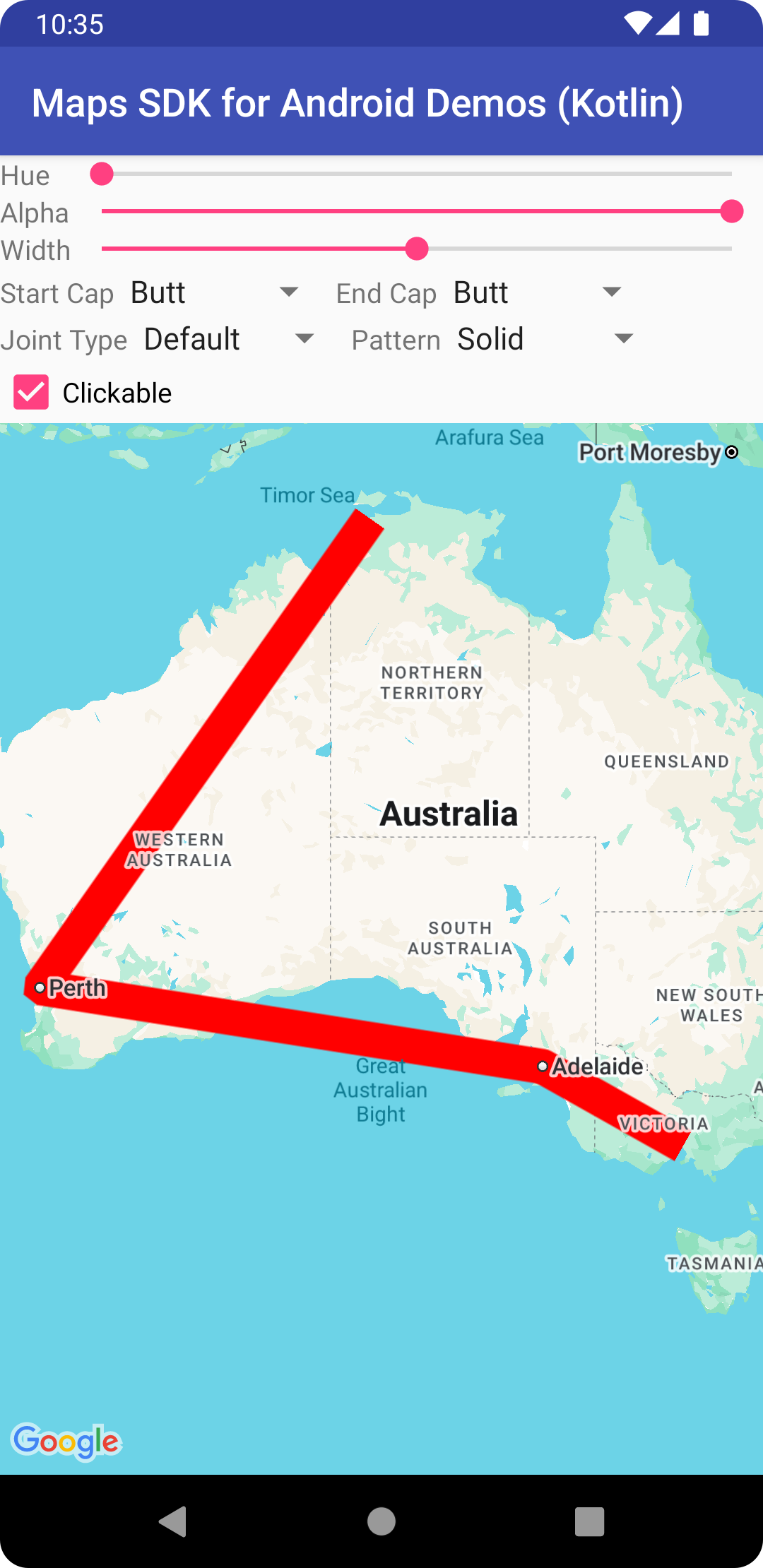Google Maps API for Android 提供一些簡單的做法，方便您在地圖中加入形狀，以根據應用程式進行自訂。

## 折線

`Polyline` 類別可定義地圖上的一組連續線段。`Polyline` 物件由一組 `LatLng` 地點組成，可建立依序連接各個地點的一系列線段。

### Java

```
// Instantiates a new Polyline object and adds points to define a rectangle
PolylineOptions polylineOptions = new PolylineOptions()
.add(new LatLng(37.45, -122.0))  // North of the previous point, but at the same longitude
.add(new LatLng(37.45, -122.2))  // Same latitude, and 30km to the west
.add(new LatLng(37.35, -122.2))  // Same longitude, and 16km to the south
.add(new LatLng(37.35, -122.0)); // Closes the polyline.

// Get back the mutable Polyline
Polyline polyline = map.addPolyline(polylineOptions);

```

### Kotlin

```
// Instantiates a new Polyline object and adds points to define a rectangle
val polylineOptions = PolylineOptions()
.add(LatLng(37.45, -122.0)) // North of the previous point, but at the same longitude
.add(LatLng(37.45, -122.2)) // Same latitude, and 30km to the west
.add(LatLng(37.35, -122.2)) // Same longitude, and 16km to the south
.add(LatLng(37.35, -122.0)) // Closes the polyline.

// Get back the mutable Polyline
val polyline = map.addPolyline(polylineOptions)

```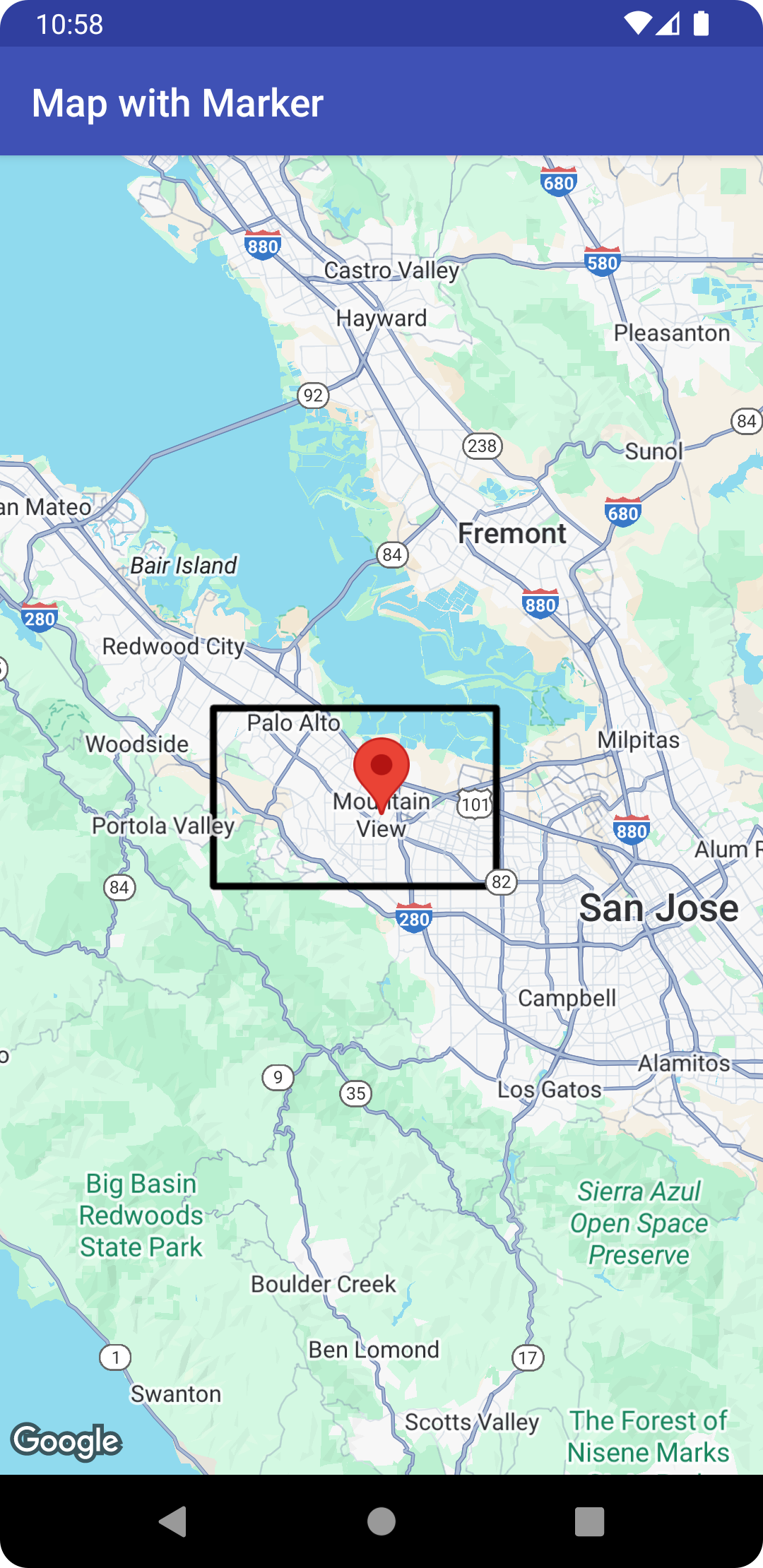## 多邊形

`Polygon` 物件與 `Polyline` 物件類似，都是由一系列座標依序組成。不過，多邊形並不是開放的線段，而是用來定義內部已填滿的封閉區域。

### Java

```
// Instantiates a new Polygon object and adds points to define a rectangle
PolygonOptions polygonOptions = new PolygonOptions()
new LatLng(37.45, -122.0),
new LatLng(37.45, -122.2),
new LatLng(37.35, -122.2),
new LatLng(37.35, -122.0));

// Get back the mutable Polygon
Polygon polygon = map.addPolygon(polygonOptions);

```

### Kotlin

```
// Instantiates a new Polygon object and adds points to define a rectangle
val rectOptions = PolygonOptions()
LatLng(37.35, -122.0),
LatLng(37.45, -122.0),
LatLng(37.45, -122.2),
LatLng(37.35, -122.2),
LatLng(37.35, -122.0)
)

// Get back the mutable Polygon
val polygon = map.addPolygon(rectOptions)

```

### Java

```
Polygon polygon1 = map.addPolygon(new PolygonOptions()
new LatLng(0, 5),
new LatLng(3, 5),
new LatLng(0, 0))
.strokeColor(Color.RED)
.fillColor(Color.BLUE));

Polygon polygon2 = map.addPolygon(new PolygonOptions()
new LatLng(0, 5),
new LatLng(3, 5))
.strokeColor(Color.RED)
.fillColor(Color.BLUE));

```

### Kotlin

```
val polygon1 = map.addPolygon(
PolygonOptions()
LatLng(0.0, 0.0),
LatLng(0.0, 5.0),
LatLng(3.0, 5.0),
LatLng(0.0, 0.0)
)
.strokeColor(Color.RED)
.fillColor(Color.BLUE)
)
val polygon2 = map.addPolygon(
PolygonOptions()
LatLng(0.0, 0.0),
LatLng(0.0, 5.0),
LatLng(3.0, 5.0)
)
.strokeColor(Color.RED)
.fillColor(Color.BLUE)
)

```

### Java

```
List<LatLng> hole = Arrays.asList(new LatLng(1, 1),
new LatLng(1, 2),
new LatLng(2, 2),
new LatLng(2, 1),
new LatLng(1, 1));
Polygon hollowPolygon = map.addPolygon(new PolygonOptions()
new LatLng(0, 5),
new LatLng(3, 5),
new LatLng(3, 0),
new LatLng(0, 0))
.fillColor(Color.BLUE));

```

### Kotlin

```
val hole = listOf(
LatLng(1.0, 1.0),
LatLng(1.0, 2.0),
LatLng(2.0, 2.0),
LatLng(2.0, 1.0),
LatLng(1.0, 1.0)
)
val hollowPolygon = map.addPolygon(
PolygonOptions()
LatLng(0.0, 0.0),
LatLng(0.0, 5.0),
LatLng(3.0, 5.0),
LatLng(3.0, 0.0),
LatLng(0.0, 0.0)
)
.fillColor(Color.BLUE)
)

```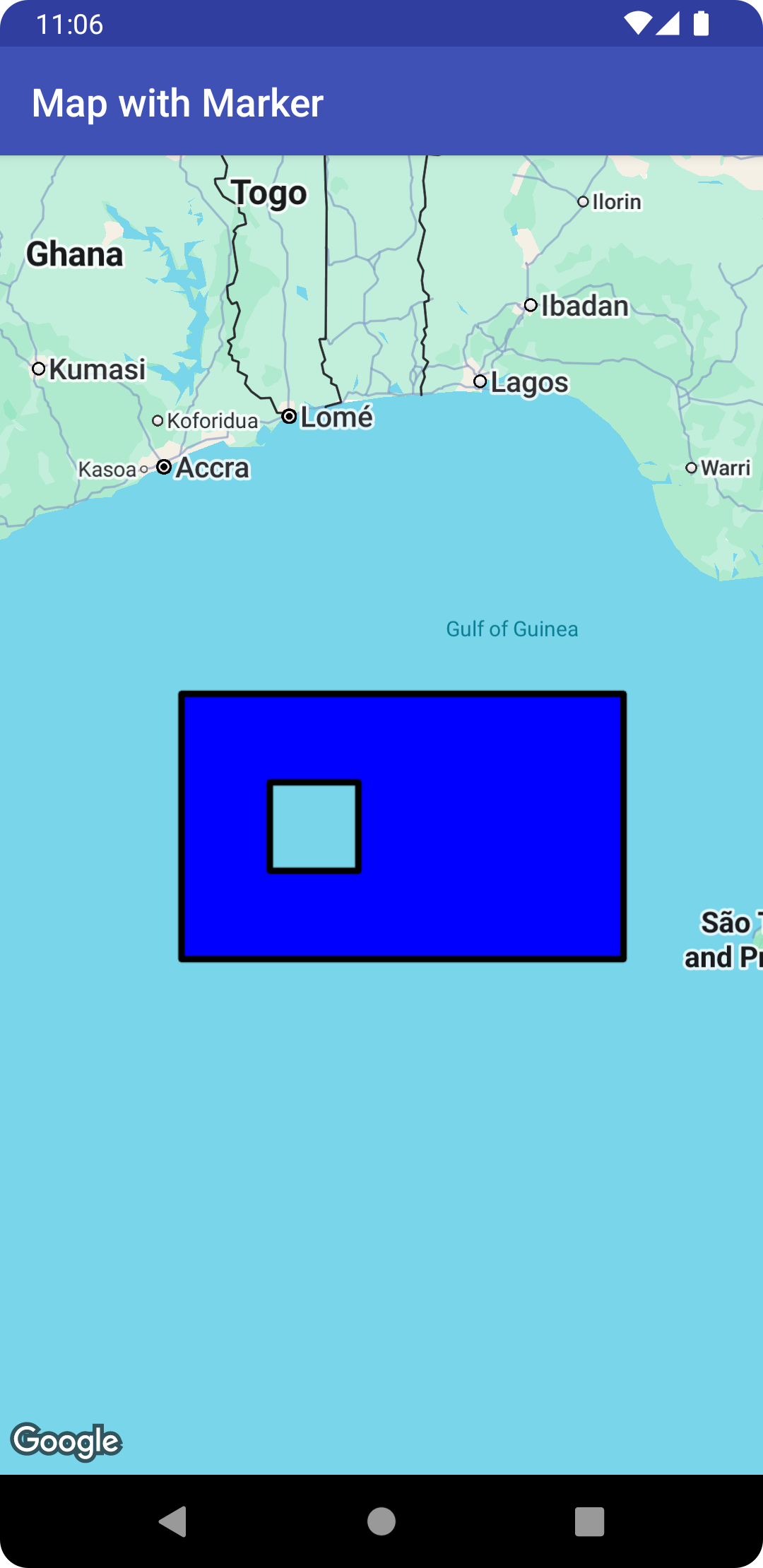## 圓形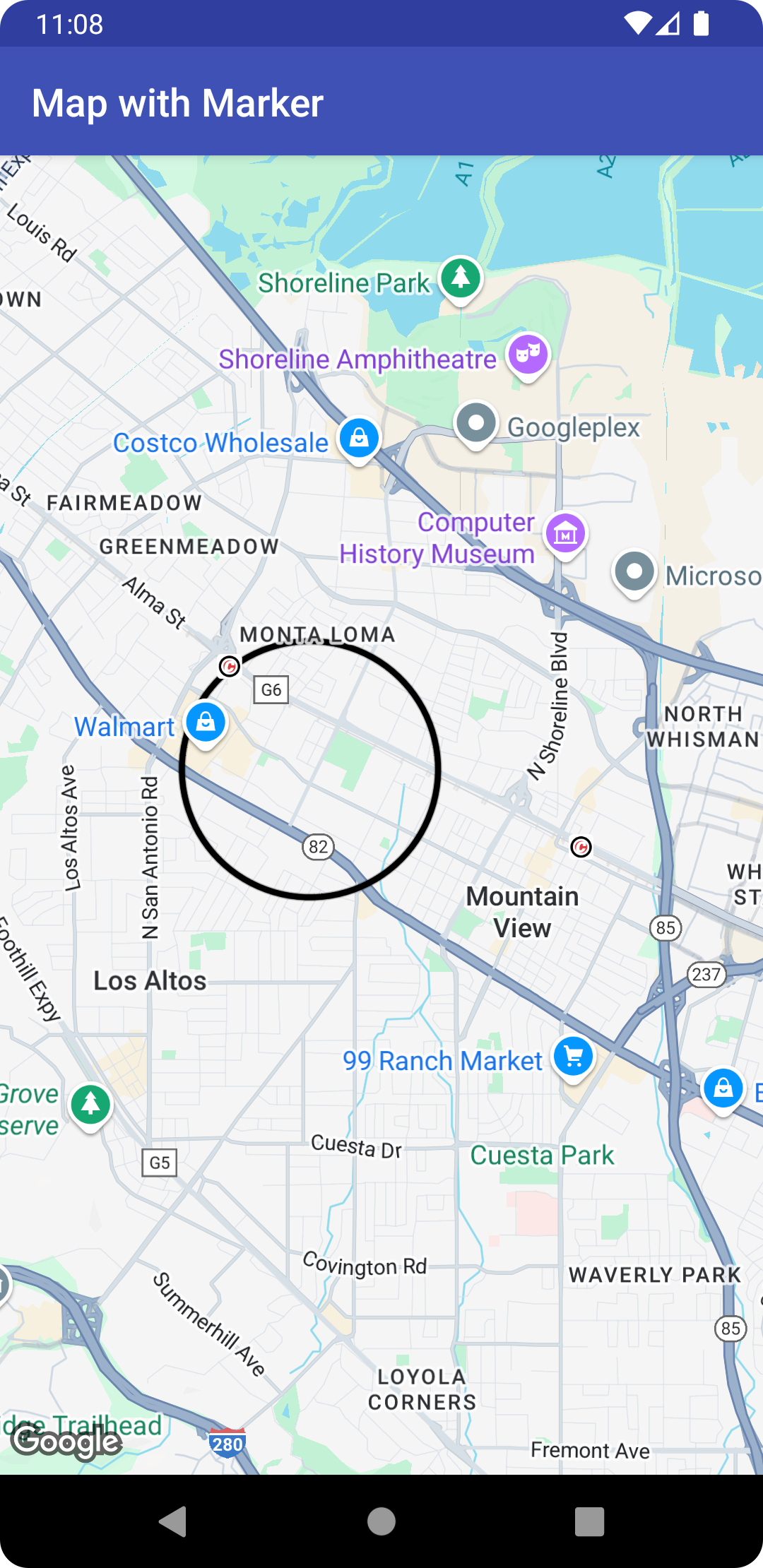• `LatLng` 格式指定的 `center`
• `radius` (單位為公尺)。

### Java

```
// Instantiates a new CircleOptions object and defines the center and radius
CircleOptions circleOptions = new CircleOptions()
.center(new LatLng(37.4, -122.1))
.radius(1000); // In meters

// Get back the mutable Circle
Circle circle = map.addCircle(circleOptions);

```

### Kotlin

```
// Instantiates a new CircleOptions object and defines the center and radius
val circleOptions = CircleOptions()
.center(LatLng(37.4, -122.1))
.radius(1000.0) // In meters

// Get back the mutable Circle
val circle = map.addCircle(circleOptions)

```

### Java

```
Circle circle = map.addCircle(new CircleOptions()
.center(new LatLng(37.4, -122.1))
.strokeWidth(10)
.strokeColor(Color.GREEN)
.fillColor(Color.argb(128, 255, 0, 0))
.clickable(true));

@Override
public void onCircleClick(Circle circle) {
// Flip the r, g and b components of the circle's stroke color.
int strokeColor = circle.getStrokeColor() ^ 0x00ffffff;
circle.setStrokeColor(strokeColor);
}
});

```

### Kotlin

```
val circle = map.addCircle(
CircleOptions()
.center(LatLng(37.4, -122.1))
.strokeWidth(10f)
.strokeColor(Color.GREEN)
.fillColor(Color.argb(128, 255, 0, 0))
.clickable(true)
)
map.setOnCircleClickListener {
// Flip the r, g and b components of the circle's stroke color.
val strokeColor = it.strokeColor xor 0x00ffffff
it.strokeColor = strokeColor
}

```

## 自訂外觀

### Java

```
Polyline polyline = map.addPolyline(new PolylineOptions()
.add(new LatLng(-37.81319, 144.96298), new LatLng(-31.95285, 115.85734))
.width(25)
.color(Color.BLUE)
.geodesic(true));

```

### Kotlin

```
val polyline = map.addPolyline(
PolylineOptions()
.add(LatLng(-37.81319, 144.96298), LatLng(-31.95285, 115.85734))
.width(25f)
.color(Color.BLUE)
.geodesic(true)
)

```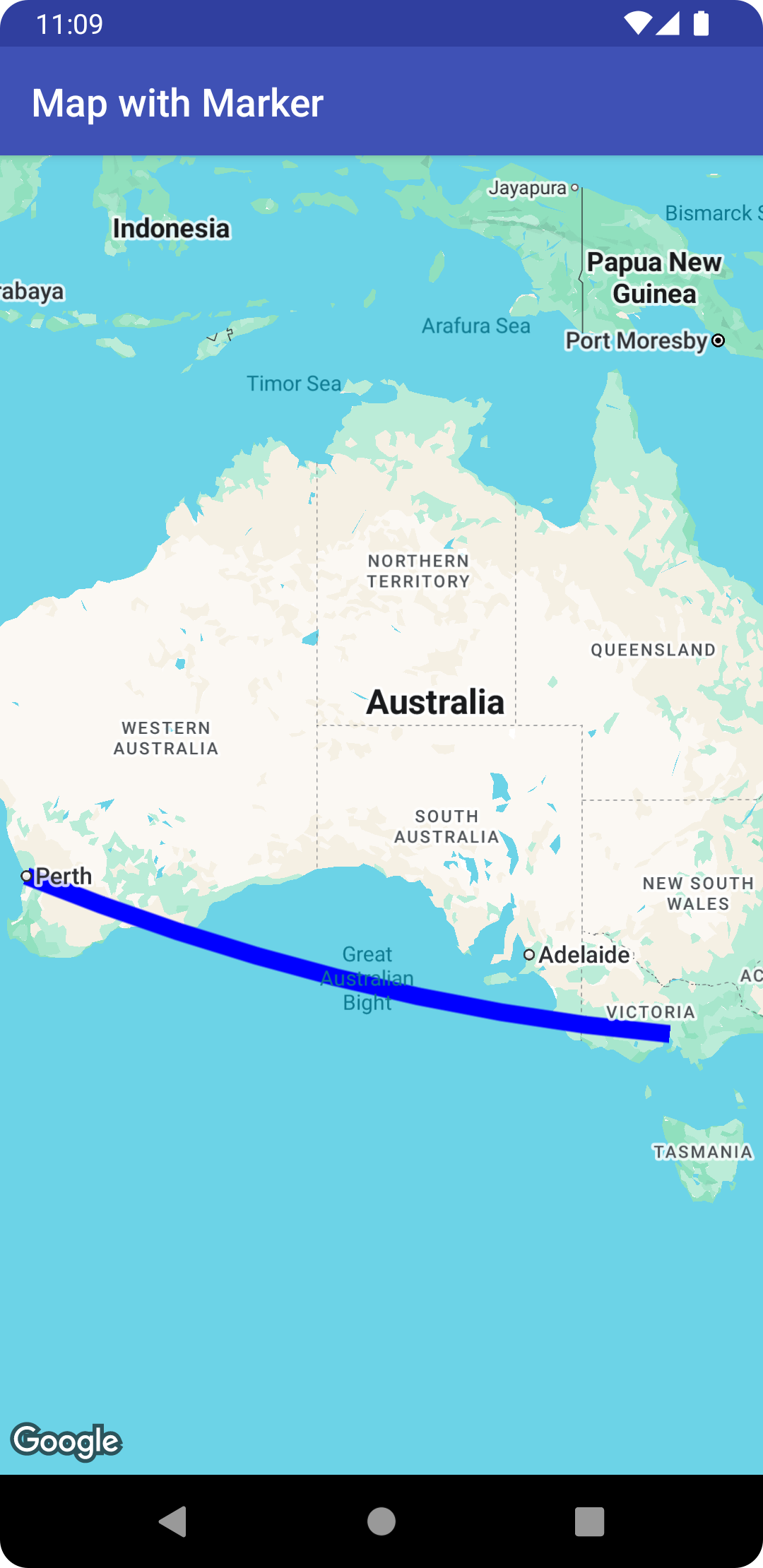### Java

```
List<PatternItem> pattern = Arrays.asList(
new Dot(), new Gap(20), new Dash(30), new Gap(20));
polyline.setPattern(pattern);

```

### Kotlin

```
val pattern = listOf(
Dot(), Gap(20F), Dash(30F), Gap(20F)
)
polyline.pattern = pattern

```

### Java

```
polyline.setJointType(JointType.ROUND);

```

### Kotlin

```
polyline.jointType = JointType.ROUND

```

### Java

```
polyline.setStartCap(new RoundCap());

```

### Kotlin

```
polyline.startCap = RoundCap()

```

### Java

```
polyline.setEndCap(
new CustomCap(BitmapDescriptorFactory.fromResource(R.drawable.arrow), 16));

```

### Kotlin

```
polyline.endCap = CustomCap(BitmapDescriptorFactory.fromResource(R.drawable.arrow), 16F)

```

### Z-index

Z-index 會指定這個形狀相對於地圖上其他疊加層 (其他形狀、區域疊加層和圖塊疊加層) 的堆疊順序。系統會將 Z-index 值較高的疊加層繪製在 Z-index 值較低的疊加層上；若兩個疊加層的 Z-index 值相同，則由系統任意決定繪製順序。

## 為資料與形狀建立關聯

### Java

```
Polyline polyline = map.addPolyline((new PolylineOptions())
.clickable(true)
new LatLng(-34.747, 145.592),
new LatLng(-34.364, 147.891),
new LatLng(-33.501, 150.217),
new LatLng(-32.306, 149.248),
new LatLng(-32.491, 147.309)));

polyline.setTag("A");

```

### Kotlin

```
val polyline = map.addPolyline(
PolylineOptions()
.clickable(true)
LatLng(-35.016, 143.321),
LatLng(-34.747, 145.592),
LatLng(-34.364, 147.891),
LatLng(-33.501, 150.217),
LatLng(-32.306, 149.248),
LatLng(-32.491, 147.309)
)
)
polyline.tag = "A"

```

• 應用程式支援不同類型的形狀，而您想要在使用者點擊形狀時，分別以不同的方式處理。
• 您操作的系統可能採用不重複的記錄 ID，而在該系統內，形狀代表特定記錄。
• 您可利用形狀資料指出優先順序，藉此決定形狀的 Z-index。
[{ "type": "thumb-down", "id": "missingTheInformationINeed", "label":"缺少我需要的資訊" },{ "type": "thumb-down", "id": "tooComplicatedTooManySteps", "label":"過於複雜/步驟過多" },{ "type": "thumb-down", "id": "outOfDate", "label":"過時" },{ "type": "thumb-down", "id": "translationIssue", "label":"翻譯問題" },{ "type": "thumb-down", "id": "samplesCodeIssue", "label":"示例/程式碼問題" },{ "type": "thumb-down", "id": "otherDown", "label":"其他" }]
[{ "type": "thumb-up", "id": "easyToUnderstand", "label":"容易理解" },{ "type": "thumb-up", "id": "solvedMyProblem", "label":"確實解決了我的問題" },{ "type": "thumb-up", "id": "otherUp", "label":"其他" }]